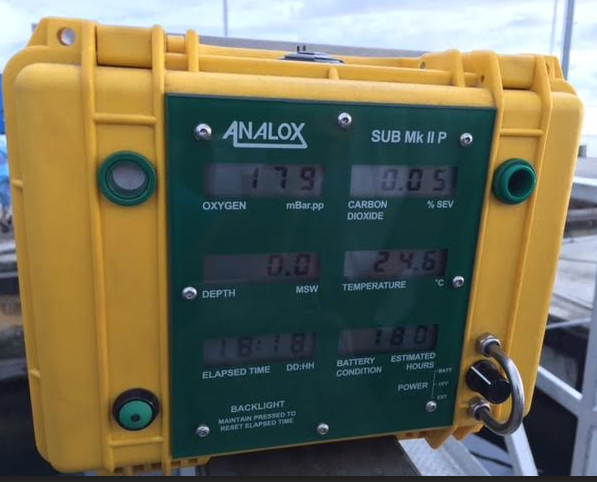We received a query on how to convert the reading of oxygen on the Analox Sub MK II P from mBar.pp to %

We were provided with the following picture:Using the values in the image provided:

%O2 = [mBar.pp O2 / Absolute pressure (mBar)] x 100%

Absolute Pressure (mBar) = (MSW +10) x 100

So %O2 = [mBar.pp O2] / [MSW + 10]

= 179 / 10

= 17.9% O2

If a simpler solution is required, there is an excel calculator attached to calculate the value for you